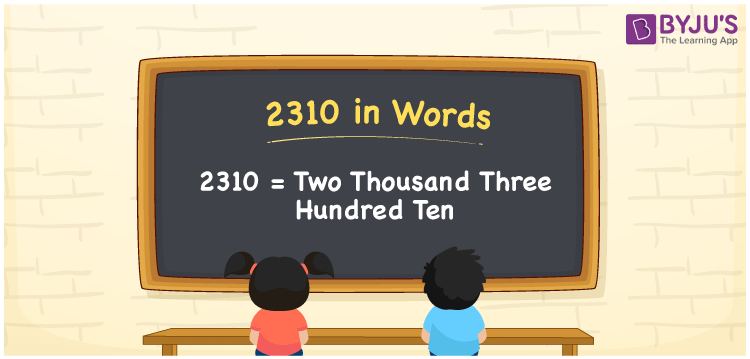# 2310 in Words

2310 in words can be written as Two Thousand Three Hundred Ten. The number name of 2310 is written considering the ones, tens, hundreds and thousands place. If you buy a cloth material for Rs. 2310 from the garments, then you can say that “I bought a cloth material for Two Thousand Three Hundred Ten Rupees from the garments”. Here you will obtain complete details of numbers in words based on the understanding abilities of students. 2310 can be read as “Two Thousand Three Hundred Ten” in English.

 2310 in words Two Thousand Three Hundred Ten Two Thousand Three Hundred Ten in Numbers 2310

## 2310 in English Words## How to Write 2310 in Words?

Find the place value of the four digits of the number 2310 from the table provided below. The expanded form is also given in a stepwise manner to improve the conceptual knowledge among students.

 Thousands Hundreds Tens Ones 2 3 1 0

2310 in expanded form can be written as:

2 x Thousand + 3 × Hundred + 1 × Ten + 0 × One

= 2 x 1000 + 3 x 100 + 1 x 10 + 0 x 1

= 2000 + 300 + 10

= 2310

= Two Thousand Three Hundred Ten

Hence, 2310 in words is written as Two Thousand Three Hundred Ten.

2310 is a natural number that precedes 2311 and succeeds 2309.

2310 in words – Two Thousand Three Hundred Ten

Is 2310 an odd number? – No

Is 2310 an even number? – Yes

Is 2310 a perfect square number? – No

Is 2310 a perfect cube number? – No

Is 2310 a prime number? – No

Is 2310 a composite number? – Yes

## Frequently Asked Questions on 2310 in Words

Q1

### How to write 2310 in words?

2310 can be written in words as “Two Thousand Three Hundred Ten”.
Q2

### Write Two Thousand Three Hundred Ten in numbers.

Two Thousand Three Hundred Ten in numbers can be written as 2310.
Q3

### Is 2310 an even number?

2310 is an even number as it is completely divisible by 2.